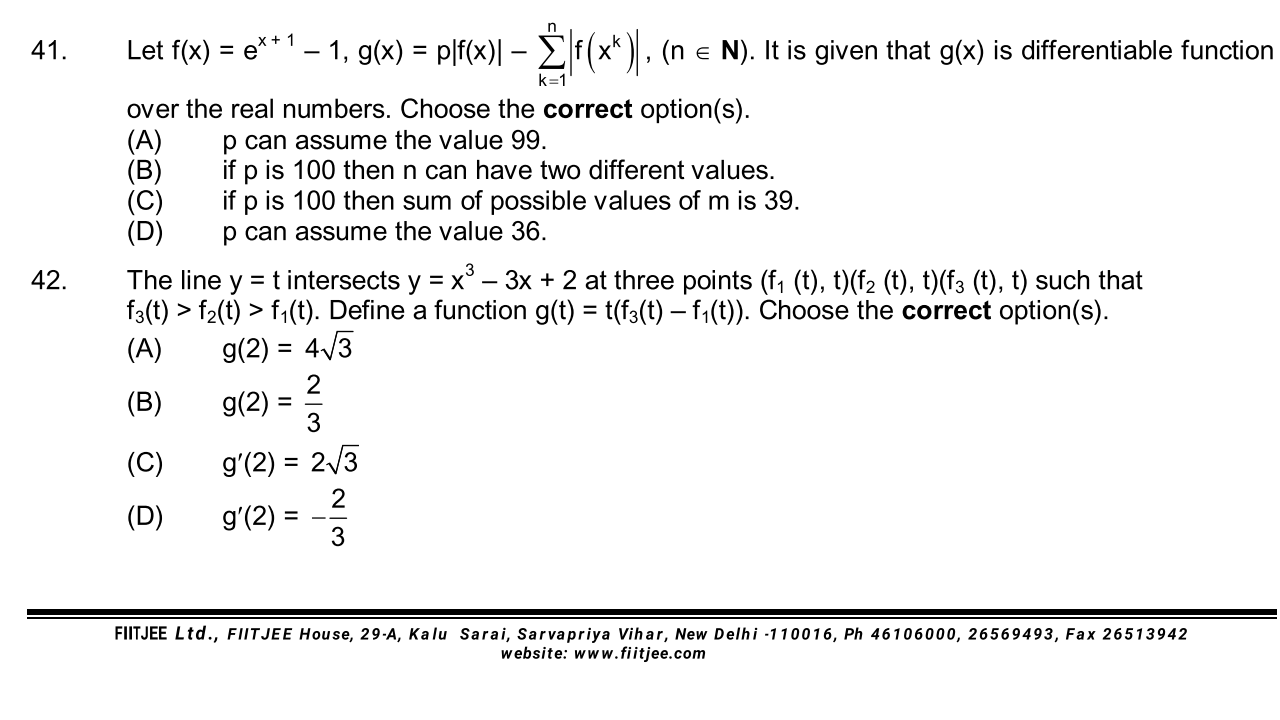# Doubt from function@Hari_Shankar sir , @Jagdish_Singh sir please share your approches for both if you have time otherwisee see 2nd problem...

For 42 I am getting A and C

share approch @Raunak_2020..

Right

Since @Raunak_2020 gave the answers i think its proper to wait for his explanation. I will certainly do so if he or any other student doesnt answer by say 8 pm

1 Like

@Hari_Shankar sir please if dont mind if you can share your approch sir..

#42: To find g(2)
x^3-3x+2=2 has roots x=-\sqrt 3, 0, \sqrt 3.

So f_1(t)=-\sqrt 3, f_3(t) = \sqrt 3 and so Option A is correct

\displaystyle g'(2) = \lim_{h \rightarrow 0}\dfrac{g(2+h)-g(2)}{h}

To find f_1(2+h) we have to solve x^2-3x+2=2+h or x^3-3x=h

Let P(x)=x^3-3x. Now P(-\sqrt 3)=0. Also \$P'(-\sqrt 3)=6

Hence we have that P\left( -\sqrt 3 + \dfrac{h}{6} \right) \approx P(-\sqrt 3)+6\times \dfrac{h}{6}=h. Hence f_1(2+h) = -\sqrt 3 + \dfrac{h}{6}

Similarly f_3(2+h) = \sqrt 3 + \dfrac{h}{6}

Hence g(2+h)-g(2) = (2+h) . 2\sqrt 3 - 4 \sqrt 3=2\sqrt 3 h

Hence \displaystyle g'(2) = \lim_{h \rightarrow 0}\dfrac{g(2+h)-g(2)}{h} = \lim_{h \rightarrow 0} \dfrac{2\sqrt 3h}{h} = 2 \sqrt 3

4 Likes

thanks hari shankar sir your approch just astounds me always..

Can you explain question 41 sir??